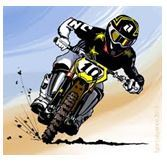Checkout JEE MAINS 2022 Question Paper Analysis : Checkout JEE MAINS 2022 Question Paper Analysis :

# Word Problems on Decimal Fractions

A fraction represents a part of whole.

For example, it tells how many slices of a pizza left or eaten with respect to the whole pizza like, one-half, three-quarters.

Generally, a fraction has two parts i.e. the numerator and the denominator. A decimal fraction is a fraction where its denominator is a power of 10 i.e. 101,102, 103 etc.

For example 32/10,56/100,325/1000. It can be expressed as a decimal like 32/10=3.2,56/100=0.56.

We can perform all arithmetic operations on fractions by expressing them as a decimal. Let’s practice some word problems on decimal fractions by using various arithmetical operations.

Example 1: A barrel has 56.32 liters capacity. If Supriya  used 21.19 liters how much water is left in the barrel.

Solution: Given,

Capacity of the barrel = 56.32 liters

Amount of water used= 21.19 liters

Amount of water left in the barrel = 56.32 – 21.19 = 35.13 liters

Example 2: Megha bought 12 bags of wheat flour each weighing 4563/100  kg. What will be the total weight?

Solution:  Total no. of bags = 12

Weight of each bag =  4563/100 kg = 45.63 kg

Total weight =45.63 x 12=547.56 kg

Example 3: If circumference of a circle is 16.09 cm. What will be its diameter(π=3.14)?

Solution: Given, circumference = 16.09 cm

Circumference of a circle, C=2πr

$$\begin{array}{l}\Rightarrow 16.09 = 2 \pi r\end{array}$$

$$\begin{array}{l}\Rightarrow 16.09 = 2 \times 3.14 \times r\end{array}$$

$$\begin{array}{l}\Rightarrow r = \frac{16.09 \times 100}{6.28 \times 100}\end{array}$$

$$\begin{array}{l}\Rightarrow r = 2.56 \end{array}$$
cm

Therefore Diameter = 2r = 2 x 2.56 = 5.12 cm.

Example 4: If the product of 38.46 and another number is 658.17, what is the other number?

Solution: Given,

One number = 38.46

Product of two numbers = 658.17

The other number = 658.17÷38.46

=

$$\begin{array}{l}\frac{658.17}{100}\div \frac{38.46}{100}\end{array}$$

= 17.11

Example 5: Rakesh bought a new.  He went on a road trip of 165.9 km on bike. After a week he went for another trip of 102.04 km. What will be the reading on meter reader of the bike?Solution: Given,

Distance travelled on first trip = 165.9 km

Distance travelled on second trip = 102.04km

Total distance travelled = 165.9 + 102.04 = 267.94 km

To solve more problems on the topic, download Byju’s – The Learning App from Google Play Store and watch interactive videos. Also, take free tests to practice for exams. To study about other topics, visit www.byjus.com and browse among thousands of interesting articles.